## River CrossingHere's the situation. You are standing on one bank of a river. You want to get to the point exactly opposite, on the other bank. You plan to do this in two stages. Stage one is to cross the river, say by swimming or rowing a boat. As you cross, you'll get swept downstream by the current. When you get to the other side, you plan to walk back upstream to your destination. Walking upstream is stage two.

It occurs to you that you might save some time by pointing upstream while crossing the river. It will take a little longer to cross the river that way, but you won't get swept downstream so far. That means less time walking back upstream, and you hope to come out ahead overall.

Does this actually work? If it does, then how much time can you save, and under what conditions? (Continue to see some answers.)

### A Quick Peek at the Answers

Just to make you curious, here are some answers:
• Yes, pointing upstream can help. (You guessed that, right?)
• But if you really are swimming, it can't help much. At typical speeds (swim at 1/2 mile/hour, walk at 3 miles/hour), then at best you can only save 1.3%. (That's right, 48 seconds per hour -- hardly worth the trouble unless you're competing in a triathlon!).
• On the other hand, if you're rowing a boat, things get a lot better. If you row the boat at 3 miles/hour, you can save as much as 23% (almost 14 minutes per hour) by pointing upstream at just the right angle. (Hhmm, maybe this is worth thinking about, after all.)
•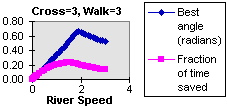And in any case -- the time that you save, and the best angle, depend on the river speed in a complicated way. If you make graphs of the best angle and the fraction of time saved, they look like this.
Why is that?? And how could you ever figure this stuff out, anyway?
(Continue to find out.)

### Making the River Crossing Graph

Believe it or not, this problem can be handled pretty well using middle-school math, plus some sophisticated features of a modern commercial "spreadsheet" program.

The general strategy is like this:

1. Break the problem into pieces that are simple enough to solve individually.
2. Write the solution to each piece as a formula. Taken together, all of these formulas will let you figure out long your trip will take, if someone specifies the river speed, the angle that you point upstream, how fast you walk, and so on.
3. Put the formulas into a spreadsheet program that can handle the numbers for you.
4. Run some test cases to check your formulas and develop your understanding.
5. Use the spreadsheet to explore lots of different conditions -- see how the time varies with river speed, angle, walking speed, and so on.
6. Think carefully about the results to decide what they mean.

### Looking at the Problem Step by Step

This problem is pretty complicated, so we're going to break it into pieces and work on the pieces one at a time. For most of the problem, we're going to end up with just 7 simple math formulas. We'll get there by starting with these questions, and working down to finer levels of detail as we need to:

When we've got the first 7 formulas in hand, we'll use them to look at the problem in several ways.

• Explore a simple swim-and-walk spreadsheet that computes total time, when you plug in values for the river speed, swim speed, swim angle, and so on.
• How does total time depend on the swim angle? (Plug values into the spreadsheet.)
• Find the best swim angle by trial and error.
• How can you use Excel's add-in "solver" to automatically find the best swim angle?
• How far you can be from the best swim angle and still get most of the benefit?
• How does the best swim angle change depending on river speed, walk speed, swim speed, etc.
• What if you don't have Excel's solver -- can you write your own program to do the same thing?
• What more can you find out by using calculus? (It turns out that there's a simple formula for the best swim angle. Getting the formula is not so simple, though!)
• How do the numerical results compare with the predictions of the formula from calculus?

Now, on to the math!

### How Long Does It Take to Cross the River?

Many "how long?" problems can be solved easily using Time=Distance/Speed or some equivalent formula. For example, this formula would be easy to apply if you were going straight across a lake.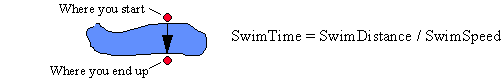But it may not be so easy to see how this formula applies to the problem of swimming (or canoeing) at some angle across a river. There are two difficulties:

### How Does Swim Angle Affect Your Time to Cross the River?

For a moment, let's imagine that the water in the river is not moving. (That is, the river acts like a long skinny lake.) Then as you swim across the river at an angle, this is what happens.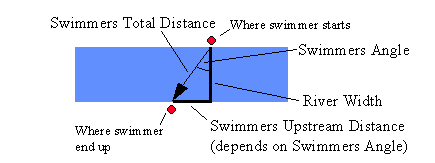Now, whatever the value of Swimmers Total Distance is, we know that
Swim Time = Swimmers Total Distance / Swimmers Speed
by the standard formula. Swimmers Total Distance, Upstream Swimmers Distance, and Swimmers Angle are interdependent -- if you specify any one, plus RiverWidth, you can calculate the other two.
Continue, or jump ahead to see what is the effect of the water moving.

### Calculating Swimmers Total Distance

Since you may not know any trigonometry yet, let's proceed by specifying Swimmers Upstream Distance. Then we can compute Swimmers Total Distance using the Pythagorean Theorem. In the notation used by Excel (and many other computer programs), this is

SwimmersTotalDistance = sqrt(RiverWidth^2 + SwimmersUpstreamDistance^2) Continue to see how to calculate Swimmers Angle, or jump ahead to see what is the effect of the water moving.

### Calculating Swimmers Angle

Well, there's no avoiding it -- now we need some trigonometry!

But that's OK -- the pieces we need are simple, and you'll be needing them often anyway. What we have here is a typical application -- find the angles of a right triangle when you know the lengths of its sides.

You can go review the basic trigonometry functions to see how they work in general. The one we need here is arcsine (spelled "asin" in Excel and most other computer languages). This lets us find an angle when we know the lengths of the hypotenuse and the side opposite the angle.

SwimmersAngle = asin(SwimmersUpstreamDistance / SwimmersTotalDistance)

The final catch is that we need to be careful about the units that we to measure angles. In math. including most calculators and spreadsheets, angles are usually measured radians. But if you prefer degrees, just multiply radians by 180/pi. (Next: what is the effect of the water moving?)

### What Is the Effect of the Water Moving?

The key to solving this problem is to imagine that you're swimming across a block of water that's part of the river. As the block of water moves down the river, it carries you with it, but that doesn't affect your swim with respect to the block of water.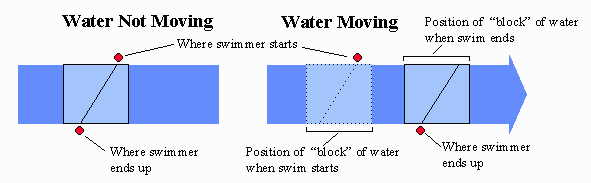So, your time to cross the river is the same as if the river were not flowing, even though you may drift quite a ways downstream.
(Continue)

### What is the Swim Time When the Water Moves?

As explained above, Swim Time is not affected by River Speed once you have picked Swimmers Angle or Swimmers Upstream Distance. So, the formula is simple:

Swim Time = Swimmers Total Distance / Swim Speed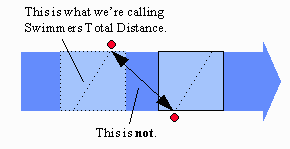Just remember, though -- this distance and speed refer to the water that the swimmer moves through. They do not include the extra distance and speed due to the swimmer being swept along with the block of water.

### How Far Do You End Up Downstream?

Now we're all set to figure out how far you end up downstream.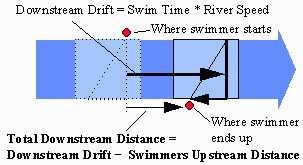The strategy is to figure out far the block of water drifts downstream, by using the River Speed and the Swim Time (which we calculated earlier). Then we subtract the Swimmers Upstream Distance (remember, this is measured relative to the block of water), from the block of water's Downstream Drift.

This difference is your Total Downstream Distance, which is how far you have to walk back upstream.

### How Much Time Do You Walk, and What's Your Total Travel Time?

Whew -- after all the hard work getting this far, these questions are easy!

For the first part, all we have to do is time = distance / speed:

Walk Time = Total Downstream Distance / Walk Speed

And then of course your total time is just the time you spent swimming, added to the time you spent walking:

Total Time = Swim Time + Walk Time

Wasn't that a refreshing break?

### All the Formulas at Once

Well, it's been a long discussion, so we shouldn't be surprised to find that we've ended up with quite a few formulas. Sure enough, there are 7 of them:

SwimmersTotalDistance = sqrt(RiverWidth^2 + SwimmersUpstreamDistance^2)
Swim Time = Swimmers Total Distance / Swim Speed
SwimmersAngle = asin(SwimmersUpstreamDistance / SwimmersTotalDistance)
Downstream Drift = Swim Time * River Speed
Total Downstream Distance = Downstream Drift - SwimmersUpstreamDistance
Walk Time = Total Downstream Distance / Walk Speed
Total Time = Swim Time + Walk Time

When all these formulas get crammed together like this, they look complicated.

### Review of the Basic Ideas

Each of our formulas came from just one application of some simple idea:
1) Pythagorean theorem,
2) time = distance / speed,
3) distance = time * speed,
4) sums and differences of distances, and
5) the arcsine function for right triangles (which we didn't really need to solve the problem, just to get the angle expressed in a familiar way).

Simple ideas, applied one at a time. The end result looks complicated, but it's just a bunch of simple ideas applied one after another.

### Crosscheck the Formulas by Plugging in Numbers

When you derive formulas, it's always a good idea to plug in numbers, run through the formulas "by hand", and see if the results make sense. If they do, then there's a good chance that you got the formulas right. And if they don't, well, back to the drawing board! Maybe you made a mistake in deriving the formula, or maybe you evaluated wrong, or maybe your idea of "making sense" is wrong. They all happen, and frequently -- at least in the author's experience!

These "numerical checks" are very important. It's easy to make mistakes in deriving formulas, and even easier to overlook the mistakes when you check the math later. But if you plug in numbers, and the numbers don't make sense, errors in the formulas are likely to become obvious.

We think our formulas are correct, but you really ought to haul out a calculator and run through them to be sure. For example, if you plug in River Speed = 3, River Width = 0.1, Swim Speed = 0.5, Walk Speed = 3, and Swimmers Upstream Distance = 0.02, then you ought to end up with Total Time = 0.4013 . (We'll let you figure out what the other answers are!)